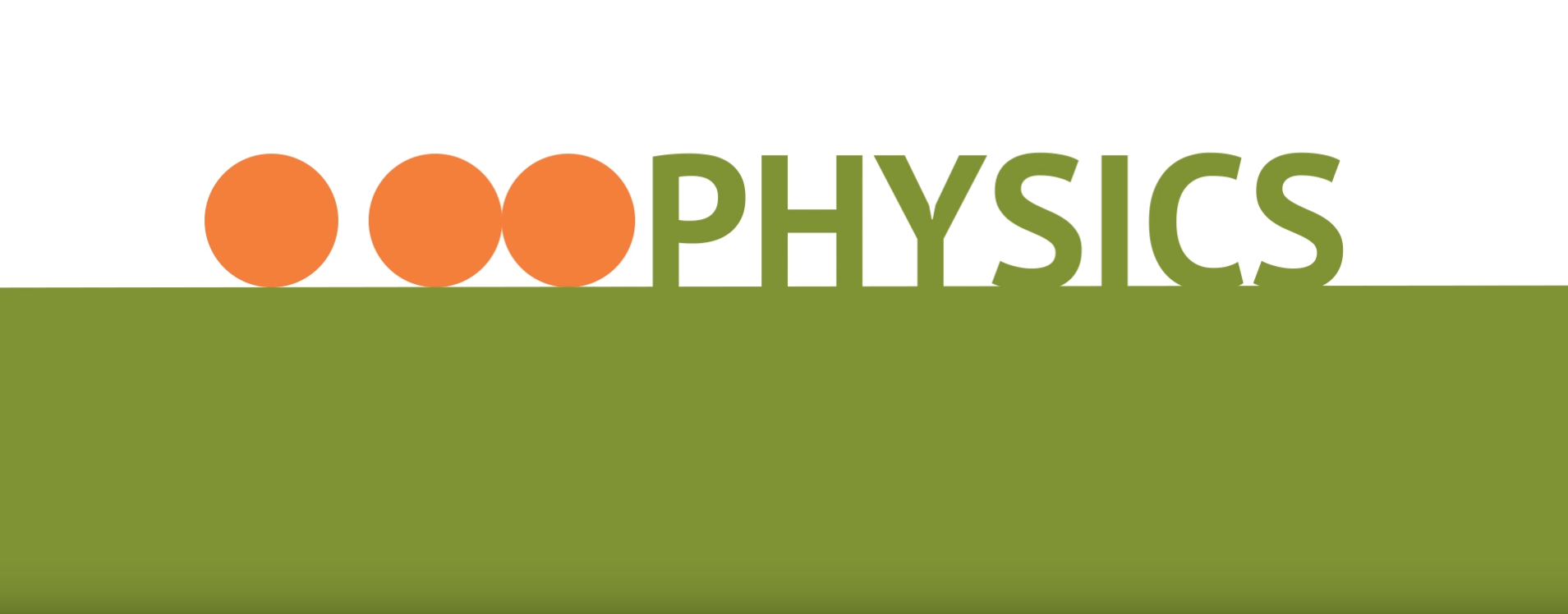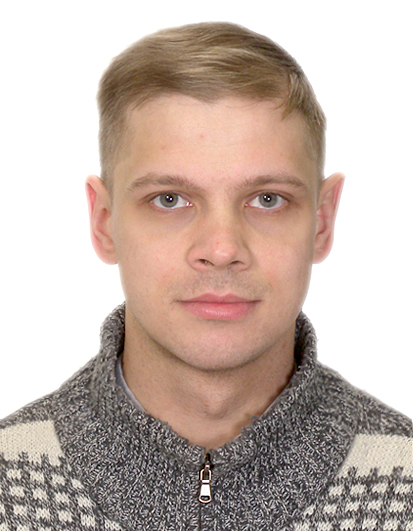# Basic physics concepts

St. Petersburg Electrotechnical University 'LETI'
Enrollment in this course is by invitation only## About the Course

This course is survey of major concepts, methods, and applications of physics. Topics include a description of motion, Newton's Laws, conservation principles (energy and momentum), waves, thermodynamics, electricity, magnetism, optics, and modern physics. It is for students who need a basic understanding of physics.

The online course is developed for use in distance and blended learning.

## Objectives

• The study of the basics of the information theory, the algebra of logic, number systems, the theory of algorithms, the basic principles of building a computer and the basics of programming.
• Formation of the ability of using computers to solve the most common problems of data processing.

## Common Course Outline

• Module 1. Mechanics
• Lesson 1.1 The main problem of mechanics
• Lesson 1.2 Newton's laws
• Lesson 1.3 Work and Energy
• Lesson 1.4 Impulse and Momentum
• Lesson 1.5 Impulse
• Lesson 1.6 Implementation of the second Newton’s law
• Lesson 1.7 The Conservation of Angular Momentum
• Lesson 1.8 Newtonian Gravitation
• Lesson 1.9 Kepler’s Laws of Planetary Motion
• Lesson 1.10 Static equilibrium
• Lesson 1.11 Friction
• Lesson 1.12 Applications of viscosity
• Lesson 1.13 Simple harmonic motion
• Module 2. Gases
• Lesson 2.1 Change of State in gas
• Lesson 2.2 Ideal Gas Equation and Absolute Temperature
• Lesson 2.3 Processes and Cycles
• Lesson 2.4 The law of energy conservation in thermodynamics
• Lesson 2.5 Second Law of Thermodynamics: Entropy and Systems
• Lesson 2.6 Thermal velocity of molecules
• Module 3. Fluids
• Lesson 3.1 Pressure Produced by Fluids
• Lesson 3.2 Archimedes’ Principle
• Lesson 3.3 Pascal’s Principle
• Lesson 3.4 Bernoulli’s Principle
• Module 4. Electricity and Electromagnetism
• Lesson 4.1 Electric force and Coulomb’s Law
• Lesson 4.2 Electric Fields & Potential
• Lesson 4.3 Electric Current
• Lesson 4.4 Power and energy in electric currents
• Lesson 4.5 Magnetism
• Lesson 4.6 Principles of Electromagnetism
• Lesson 4.7 Electromagnetic waves
• Module 5. Optics
• Lesson 5.1 Reflection and Refraction
• Lesson 5.2 Lenses and Images
• Lesson 5.3 Light waves
• Lesson 5.4 Diffraction
• Lesson 5.5 Interference
• Lesson 5.6 Polarization
• Lesson 5.7 Dispersion and Color
• Module 6. Atomic Physics
• Lesson 6.1 Blackbody radiation
• Lesson 6.2 Quantum hypothesis
• Lesson 6.3 Photoelectric effect and photons
• Lesson 6.4 Bohr model of the atom
• Lesson 6.5 Atomic spectra
• Module 7. Nuclear Physics
• Lesson 7.1 Radioactivity
• Lesson 7.2 Alpha, beta, and gamma decay
• Lesson 7.3 Nuclear binding energy
• Lesson 7.4 Nuclear fission and fusion

## Entry requirements

If you pass an introduction test for this course you will be accepted on a course.

## Technical requirements

Review the technical requirements to access the course and complete it successfully.

## Course authors### Alexander Kukaev

PhD, Associate professor of Laser Measurement and Navigation Systems Department

St.-Petersburg Electrotechnical University «LETI»### Yuriy Demidov

PhD, Associate professor of Department of Physics

St.-Petersburg Electrotechnical University «LETI»
1. Course Number

PH101
2. Classes Start

3. Classes End

4. Estimated Effort

4 hours per week
5. Number of Weeks

12